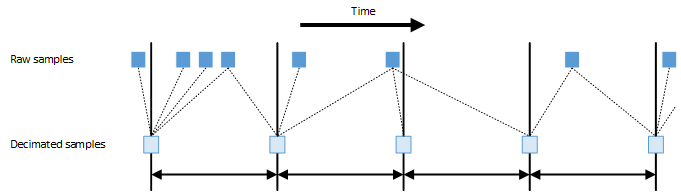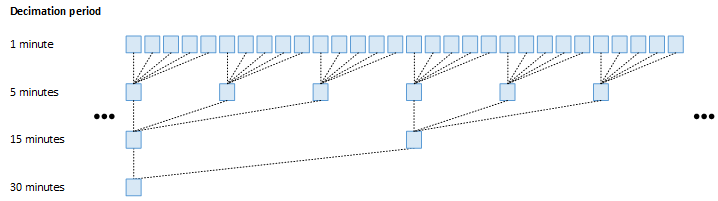## 3. Decimated samples

Users often want to retrieve samples for an extended period of time, for example in order to get a trend of how a process variable changed over months or even years. In this case, retrieving the raw samples as they were logged is rather inefficient. For example, if a process variable is logged at an update rate of one sample per second, there are 86,400 samples per day or 31,536,000 samples per year. When plotting the trend of a process variable’s value for a whole year, using 31 million samples does not make sense because the effective resolution of the plot will limit the amount of details that can be seen to a much coarser level. More importantly, retrieving the data for 31 million samples can take a considerable amount of time and typically a user will not want to wait for a long time if she is just interested in getting a quick overview.

For this reason, the Cassandra PV archiver supports so-called decimated samples. These decimated samples are generated asynchronously in the background while data is being archived. When retrieving data from the archive, this decimated data can be used when lower resolution data is sufficient for satisfying the user’s request. Decimated samples are organized in so-called decimation levels. Each decimation level for a certain channel stores samples at a fixed rate.

Typcially, the density of these decimation levels is chosen so that the distance between two samples increases exponentially with each decimation level. For example, when having a process variable with a native update rate of approximately one sample per second, the administrator might add decimation levels with decimation periods of 30 seconds, 15 minutes, and 6 hours. When plotting data for a whole year, one might then select the data from the decimation level with a decimation period of 6 hours, resulting in only 1,460 samples being returned instead of approximately 31 million raw samples.

The samples that are generated for decimation levels are always generated with a fixed distance specified by the decimation period of that decimation level. The details of how a decimated sample is generated are left to each control-system support. For example, a simple algorithm might choose to simply use one raw sample for each decimated sample, resulting in a “decimation” process in the literal sense. A more advanced algorithm, on the other hand, might choose to apply statistical operations on the source samples for the relevant period of time, calculating a mean and other stastical properties.

Figure I.3. Mapping of raw samples to decimated samplesFigure I.3, “Mapping of raw samples to decimated samples” shows how decimated samples are generated from raw samples. For each decimated sample, the Cassandra PV Archiver passes one raw sample before or at the same time as the decimated sample to be generated and all raw samples after the decimated sample but before the next decimated sample to be generated. This way, the control-system support has all relevant information for the whole period for which the decimated sample is generated. This means that a decimated sample represents the period after its time stamp. For example, when having a decimation level with a decimation period of 30 seconds, the decimated sample with a time stamp of 14:12:30 will represent the interval [14:12:30, 14:13:00).

When there are multiple decimation levels for a channel, the decimated samples for longer decimation period are generated from decimated samples from shorter decimation periods (if the longer period is an integer multiple of the shorter period). This way, the amount of data that has to be processed is reduced dramatically (see Figure I.4, “Sample generation for cascaded decimation levels”).

Figure I.4. Sample generation for cascaded decimation levels